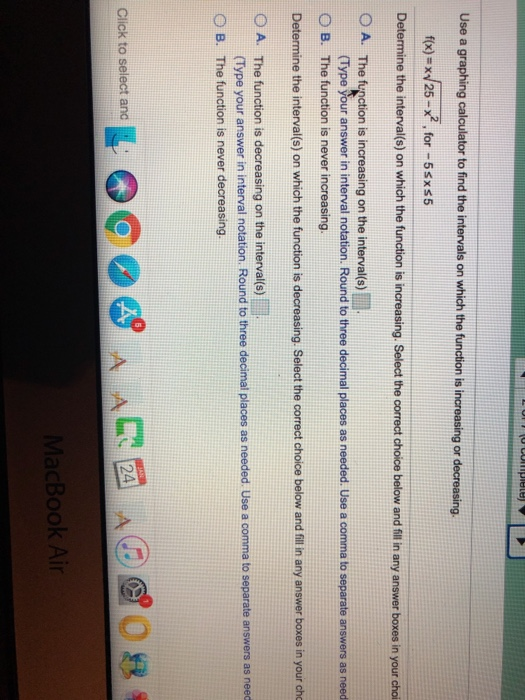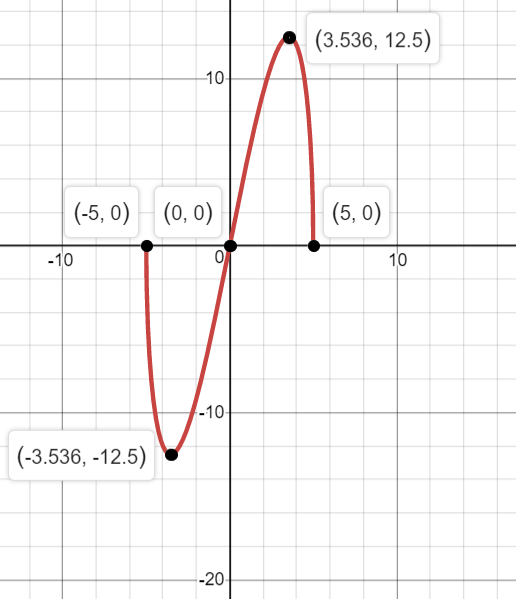# Increasing and decreasing intervals calculatorUse a graphing calculator to find the intervals on which the function is increasing or decreasing f(x)-x/25 2 , for-5sxs5 Determine the interval(s) on which the function is increasing. Select the correct choice below and fil in any answer boxes in your choi The furpction is increasing on the intervals) (Type your answer in interval notation. Round to three decimal places as needed. Use a comma to separate answers as need O B. The function is never increasing Determine the interval(s) on which the function is decreasing. Select the correct choice below and fill in any answer boxes in your cho O A. The function is decreasing on the interval(s) O B. The function is never decreasing. (Type your answer in interval notation. Round to three decimal places as needed. Use a comma to separate answers as need Click to select and 24 MacBook Air

f(x) = x sqrt ( 25 – x^2 )
graph shown below

function is increasing on
the interval ( –
3.536 , 3.536 )
function is decreasing on
the interval [ – 5
, – 3.536 ) U ( 3.536 , 5 ]